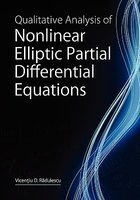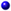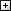Processing ......FreeComputerBooks.com Free Computer, Mathematics, Technical Books and Lecture Notes, etc.

Qualitative Analysis of Nonlinear Elliptic Partial Differential Equations
• Title Qualitative Analysis of Nonlinear Elliptic Partial Differential Equations
• Publisher: Hindawi Publishing Corporation; First edition (December 1, 2008)
• Paperback 210 pages
• Language: English
• ISBN-10: 9774540395
• ISBN-13: 978-9774540394Book Description

The book provides a comprehensive introduction to the mathematical theory of nonlinear problems described by elliptic partial differential equations. These equations can be seen as nonlinear versions of the classical Laplace equation, and they appear as mathematical models in different branches of physics, chemistry, biology, genetics and engineering and are also relevant in differential geometry and relativistic physics. Much of the modern theory of such equations is based on the calculus of variations and functional analysis.

Concentrating on single-valued or multivalued elliptic equations with nonlinearities of various types, the aim of this volume is to obtain sharp existence of nonexistence results, as well as decay rates for general classes of solutions. Many technically relevant questions are presented and analyzed in detail. A systematic picture of the most relevant phenomena is obtained for the equations under study, including bifurcation, stability, asymptotic analysis, and optimal regularity of solutions.All CategoriesRecent BooksIT Research LibraryMiscellaneous BooksComputer LanguagesComputer ScienceData Science/DatabasesElectronic EngineeringJava and Java EE (J2EE)Linux and UnixMathematicsMicrosoft and .NETMobile ComputingNetworking and CommunicationsSoftware EngineeringSpecial TopicsWeb Programming